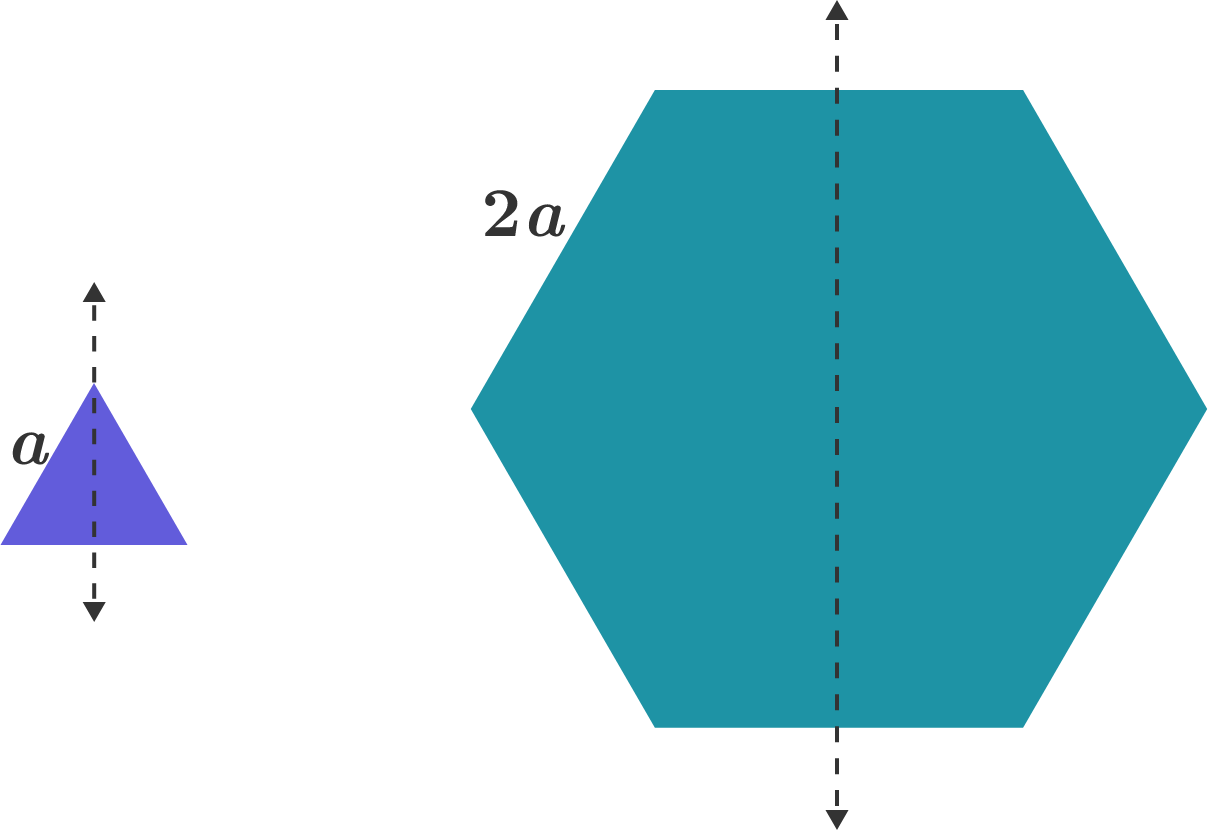# Problems of the Week

Contribute a problem

An equilateral triangular plate has side length $a$, and the moment of inertia about the axis shown is $I$.

A regular hexagonal plate has side length $2a$, and the moment of inertia about the axis shown can be given as a constant multiple of $I$: $k I$.

What is $k?$

Note: Assume both plates have negligible thickness.Let $a$ be a positive integer such that the following limit exists: $\lim_{x\to 1} \left( \frac{1}{x-1} - \frac{1}{x^a-2x+1}\right).$ If the value of the limit is $b,$ find $a+b.$

Concatenating four copies of 23 produces $23 || 23 || 23 || 23 = 23232323.$

Now, suppose you concatenate $x$ copies of any positive integer $n.$

What is the minimum value of $x$ such that the result of this concatenation is guaranteed to be a multiple of 11?

Find the number of 10-digit sequences where the difference between any two consecutive digits is 1, using only the digits 1, 2, 3, and 4.

Examples of such 10-digit sequences are 1234321232 and 2121212121.

Bonus: Let $T(n)$ be the number of such $n$-digit sequences. What is $\lim_{n \to \infty} \frac{T(n+1)}{T(n)}?$

There exist positive integers $A,B,C,D,E,F$ such that $\sum_{p=1}^n \cot^6 \left(\tfrac{p \pi}{2n+1}\right) = \tfrac{1}{F}n(2n-1)\big(An^4 + Bn^3 + Cn^2 - Dn + E\big)$ for all positive integers $n$, where $F$ is as small as possible.

What is $A + B + C + D + E + F?$

Bonus: Use this result to prove that $\sum_{p=1}^\infty \tfrac{1}{p^6} = \tfrac{1}{945}\pi^6.$

×